# Writing Custom Functions in Power Query M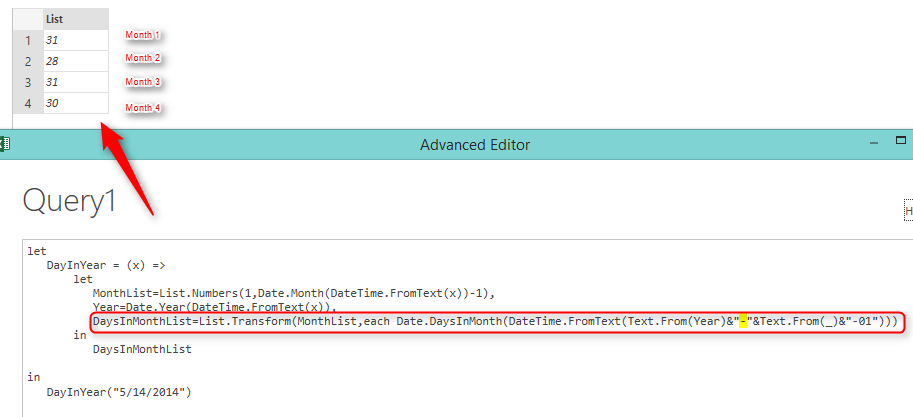One of the most powerful features of M is that you can write custom functions to re-use part of your code. Custom functions can be single or multiple liner, they will be written in Lambda style syntax. In this post you will learn how to write functions and invoke them in M.

Custom functions and some of the other features of M such as error handling, completely distinguish this language from expressions in SSIS. I’m saying this because I’ve heard questions like: Is the M similar to SSIS expressions? The answer is: definitely NO! I am a big fan of SSIS (those of you who know me would agree that), But SSIS expressions are just for calculation, and creating transformations based on expressions. Actually SSIS expressions is not a language, it is kind of an expression. M in the other hand is a language, and it is functional language, that means you don’t need to write Main function and etc, M would handle most of them simply. and you have features such as writing custom functions and error handling (You can not find most of these advanced features through Power Query GUI, So start scripting M 🙂 )

Basic Syntax

Functions in M can be written in this format:

(x) => x+1

This is lambda syntax (that previously used in LINQ if you come from .NET development background). the line above is equal to pseudo-code below:

Function anonymouss (x)

{

return x+1

}

As you see lambda made it much simpler to define the function with just that single line. So the function above get a parameter from input and adds 1 to it. Please note that datatype of parameter not defined, so that means if a text be an input, then an error would occur, so you would require to do error handling as well (I’ll describe Error Handling in M in future blog posts).

So let’s see how this function works and how we can call this function. script below shows how to define the function and invoke it with a parameter:

let
in

Result of above expression is: 11In example above we’ve named the function as "Add" and then we simply call that with Add(inputparam).

Parameters

If you want to define a function with more parameters, then simply add parameters as below:

(x,y,z) => x+y+z

you can also define a function without parameters, as below:

() => "sample function without parameter"

This function get no parameters and returns the text "sample function without parameter".Optional Parameters

parameters are defined as Required by default. that means you should specify the parameter at the time of invoking function. So if you want to define an optional parameter, use the Optional keyword as below:

let
Func = (optional x) => if x is null then "nothing" else x
in
Func(5)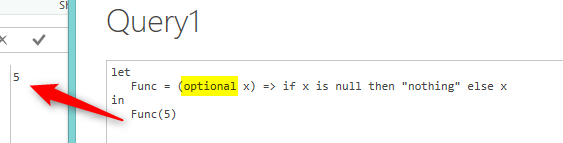Variables in Function

samples above showed how to write single line expression function, but in most of the cases you would require to write multiple line function that contains variables inside the function. in that case you can define the function within the LET / IN structure as below:

let
Func = (x) =>
let
<body>
in
<return value>
in
Func(5)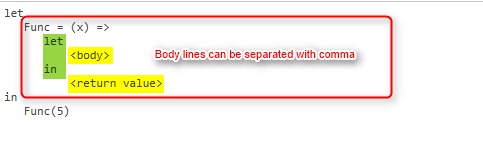As you see in the above script another set of Let/In added inside the function. you can write body of function in the LET clause. and if you want to add multiple lines there, or if you want to define variables, do this as usual with a single comma at the end of each line. Finally you can return value in the IN clause.

Example

following example show how we can use structure above to create a function that return number of days passed till a date from start of the year.

let
DayPassedInYear = (x) =>
let
MonthList=List.Numbers(1,Date.Month(DateTime.FromText(x))-1),
Year=Date.Year(DateTime.FromText(x)),
DaysInMonthList=List.Transform(MonthList,each Date.DaysInMonth(DateTime.FromText(Text.From(Year)&"-"&Text.From(_)&"-01")))
in
Date.Day(DateTime.FromText(x))+List.Sum(DaysInMonthList)

in
DayPassedInYear("5/14/2014")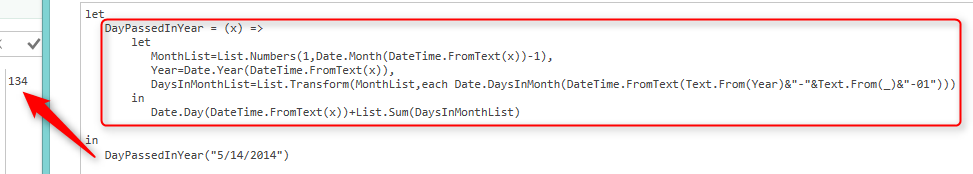Line by Line Description

Let’s go through the script line by line;

DayPassedInYear = (x) =>

In above line we define the function name as "DayPassedInYear", this function require a single parameter, definition of the body would come in next lines.

let
MonthList=List.Numbers(1,Date.Month(DateTime.FromText(x))-1),

We used Let to define a multi-line body. the first line of body is defining a variable that creates list of Month numbers from the first month (1) to the previous month of the input date. for calculating the previous month of the input date we used this expression: Date.Month(DateTime.FromText(x))-1 .

and this part generates a list based on numbers from a beginning number (1) to ending number (which would be result of previous month function): List.Numbers(1,….)

So as a result, the MonthList would be a variable that contains list of months from the first month up to prior month in the current year. Let’s see how that single line would return result: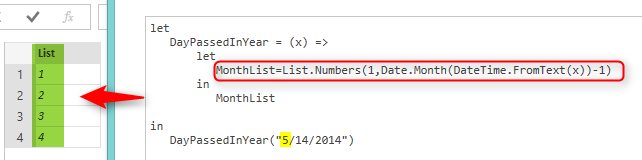Next line in the script calculates year of the input date and store that into a variable named "Year":

Year=Date.Year(DateTime.FromText(x)),

Consider that you should end each line with a single comma (if you don’t want to logic of two line to be parsed together).

The final line of the body combined from multiple expressions, I’ll describe the one by one;

DateTime.FromText(Text.From(Year)&"-"&Text.From(_)&"-01")

Above expression will return first day of a month. Please note that there is a single underscore in the expression. that underscore would be used in EACH expression. EACH is a single parameter function. we used EACH in this example to apply a transformation to every item in the list. actually we want to replace each month number in the list, with the number of days in that month. So we use EACH single liner function to fetch number of days in that month. when you use EACH, you can use underscore as the parameter marker. In simpler words if you have a list as below:

ListA

1

2

3

and if you transform that list with List.Transform function as below:

List.Transform(ListA,each _*10)

Result would be:

ListA

10

20

30

So the result of This line below:

DaysInMonthList=List.Transform(MonthList,each Date.DaysInMonth(DateTime.FromText(Text.From(Year)&"-"&Text.From(_)&"-01")))

Would be:As you see each list item which had the month number earlier, now replaced (transformed) with number of days in that month.

In the final step of the function we return the result;

in
Date.Day(DateTime.FromText(x))+List.Sum(DaysInMonthList)

IN clause used to return the result, and we use Date.Day function to return the day part of input date. and we add that with sum of all values form the list. as a result we would see number of days passed from the first of year to the input date.Summary

In this blog post, you’ve learned how to define functions and how to invoke them. you’ve learned that you can define optional or required parameters. you can also define multi-line functions that contains variables inside the body. you’ve seen a sample function that shows how useful are functions in real world. you’ve also learned about EACH keyword which can be used as a single parameter function (especially in lists and tables).

Trainer, Consultant, Mentor
Reza Rad is a Microsoft Regional Director, an Author, Trainer, Speaker and Consultant. He has a BSc in Computer engineering; he has more than 20 years’ experience in data analysis, BI, databases, programming, and development mostly on Microsoft technologies. He is a Microsoft Data Platform MVP for 12 continuous years (from 2011 till now) for his dedication in Microsoft BI. Reza is an active blogger and co-founder of RADACAD. Reza is also co-founder and co-organizer of Difinity conference in New Zealand, Power BI Summit, and Data Insight Summit.
Reza is author of more than 14 books on Microsoft Business Intelligence, most of these books are published under Power BI category. Among these are books such as Power BI DAX Simplified, Pro Power BI Architecture, Power BI from Rookie to Rock Star, Power Query books series, Row-Level Security in Power BI and etc.
He is an International Speaker in Microsoft Ignite, Microsoft Business Applications Summit, Data Insight Summit, PASS Summit, SQL Saturday and SQL user groups. And He is a Microsoft Certified Trainer.
Reza’s passion is to help you find the best data solution, he is Data enthusiast.
His articles on different aspects of technologies, especially on MS BI, can be found on his blog: https://radacad.com/blog.

## 7 thoughts on “Writing Custom Functions in Power Query M”

•Pavel says:

you are an excellent author
everything you write is very clear, logical and distinct
thank you

•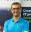Reza Rad says:

Thanks Pavel 🙂

•Babak says:

It was great Reza, like the other posts
Thanks

•Lupe says:

Great post, being quite new to M language, this really helped me!

•Jon says:

Good evening, great post. Quick question, don’t know if you or someone in your community have thought or actually done it.
Is it possible to hide the full M code of the custom function from the user. For example let’s say you create a function and save it in a repository. The function can be retrieved in a excel or power bi file using the Expression.Evaluate function by adding the url. The problem. With this method is that the urlcan be copy and paste in any browser and the full custom function file can be revealed (not so great for copyrights)
•Reza Rad says: